Question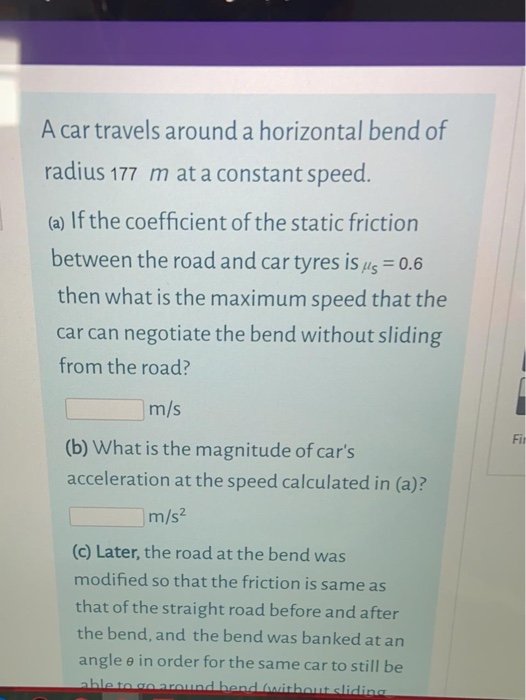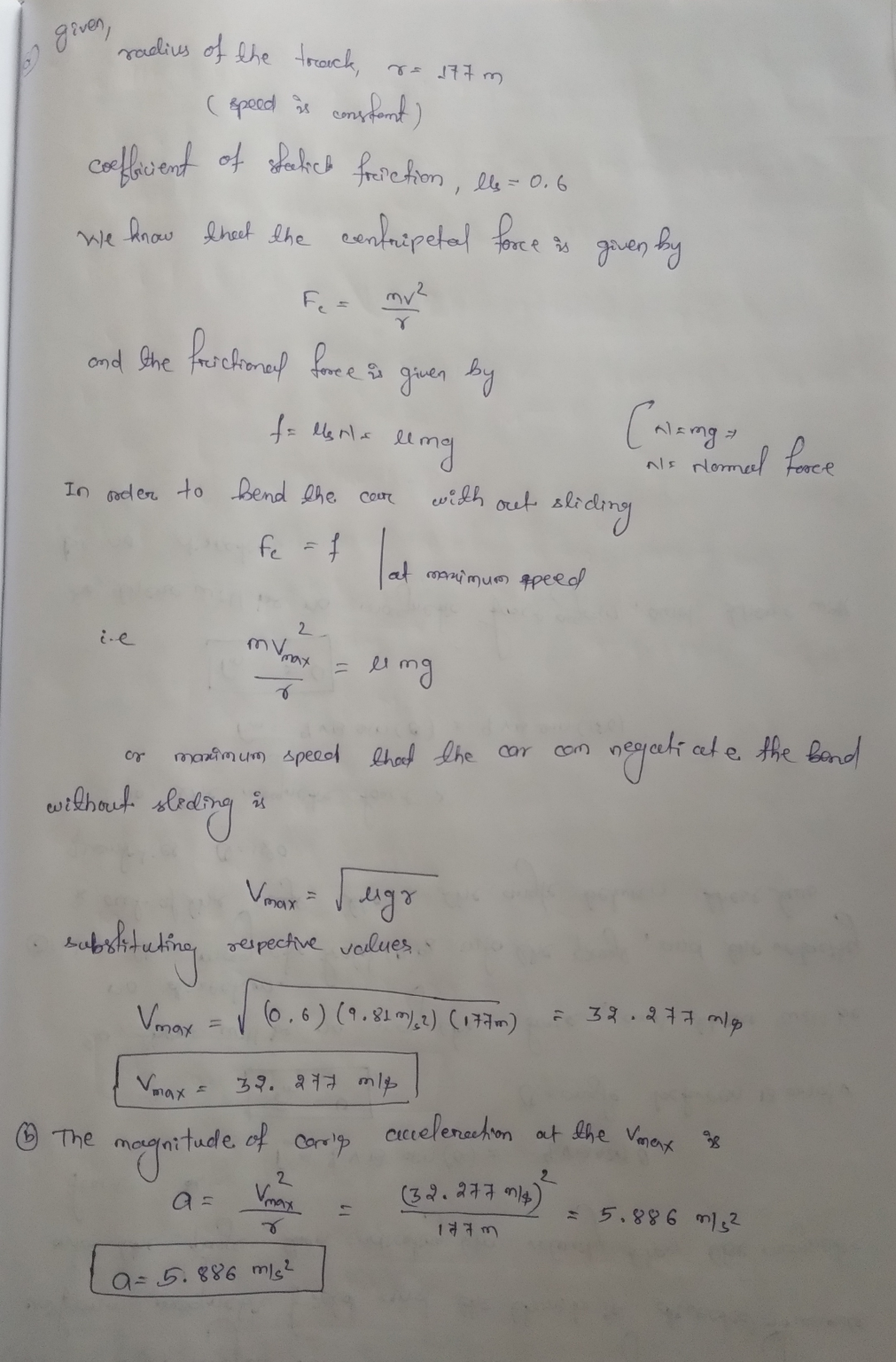Comment

A) The maximum speed of the car was found out to be Vmax=32.277 m/s.

B) the magnitude of acceleration of the car was found out to be a=5.886m/s^2

#### Earn Coins

Coins can be redeemed for fabulous gifts.

Similar Homework Help Questions
• ### A car travels at a constant speed of 32.5 mi/h (14.5 m/s) on a level circular...

A car travels at a constant speed of 32.5 mi/h (14.5 m/s) on a level circular turn of radius 49.0 m, as shown in the bird's-eye view in figure a. What minimum coefficient of static friction, μs, between the tires and the roadway will allow the car to make the circular turn without sliding? 1 ) make the circular turn without sliding? 2 ) At what maximum speed can a car negotiate a turn on a wet road with coefficient...

• ### Problem A car travels at a constant speed of 29.5 mi/h (13.2 m/s) on a level...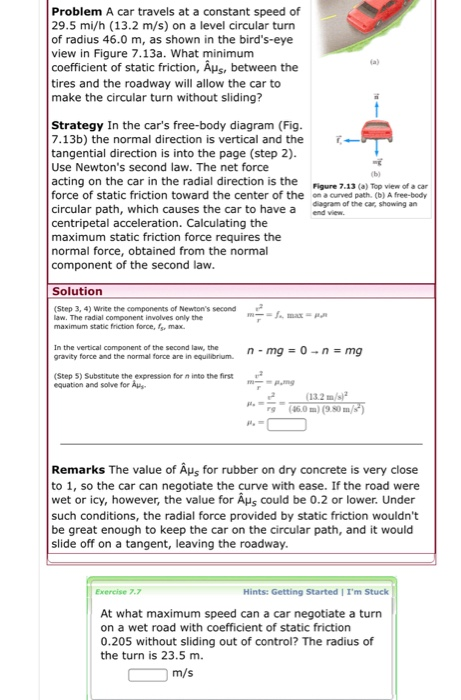Problem A car travels at a constant speed of 29.5 mi/h (13.2 m/s) on a level circular turn of radius 46.0 m, as shown in the bird's-eye view in Figure 7.13a. What minimum coefficient of static friction, Aus, between the tires and the roadway will allow the car to make the circular turn without sliding? Strategy In the car's free-body diagram (Fig. 7.13b) the normal direction is vertical and the tangential direction is into the page (step 2). Use Newton's...

• ### A car travels at constant speed around a corner. The cars speed is 35 m/s and...

A car travels at constant speed around a corner. The cars speed is 35 m/s and the radius of the circle is 0.25 km. The coefficient of static friction between the tires and the road is 0.7. What is the frictional force needed for the car to make the turn? What is the maximum force the static friction can produce? Does the car stay on the road? The car is in motion so why is the static friction important?

• ### A car travels around an unbanked 60 m radius curve without skidding, If the coefficient of...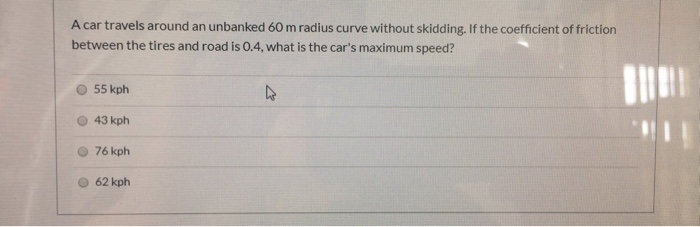A car travels around an unbanked 60 m radius curve without skidding, If the coefficient of friction between the tires and road is 0.4, what is the car's maximum speed? 55 kph 47 43 kph 76 kph 62 kph

• ### 1. A car travels at constant speed around a horizontal circular corner of radius 5 m....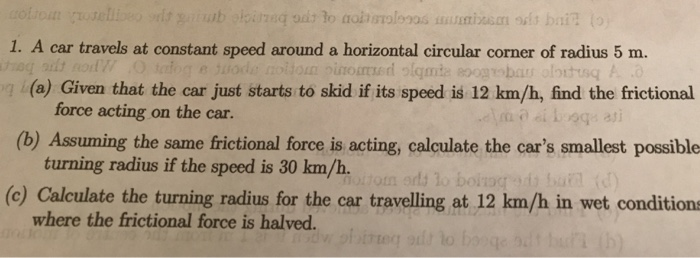1. A car travels at constant speed around a horizontal circular corner of radius 5 m. n (a) Given that the car just starts to skid if its speed is 12 km/h, find the frictional force acting on the car. (b) Assuming the same frictional force is acting, calculate the car's smallest possible turning radius if the speed is 30 km/h. (c) Calculate the turning radius for the car travelling at 12 km/h in wet conditions where the frictional force...

• ### horizontal curve

A car travels at a steady 40.0 m/s around a horizontal curve of radius 200 m. What is the minimum coefficient of static friction between the road and the car's tiresthat will allow the car to travel at this speed without sliding?choose one answer.1.230.6620.7360.9520.816

• ### A car of mass M = 800 kg traveling at 55.0 km/hour enters a banked turn...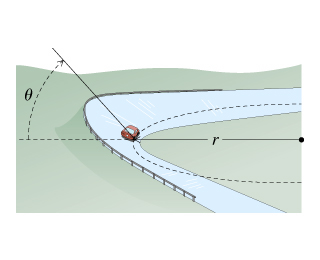A car of mass M = 800 kg traveling at 55.0 km/hour enters a banked turn covered with ice. The road is banked at an angle ?, and there is no friction between the road and the car's tires as shown in(Figure 1) . Use g = 9.80 m/s2 throughout this problem. Now, suppose that the curve is level (?=0) and that the ice has melted, so that there is a coefficient of static friction ? between the road and...

• ### A 1080 kg Smart car is going around a 58 m radius curve. The coefficient of...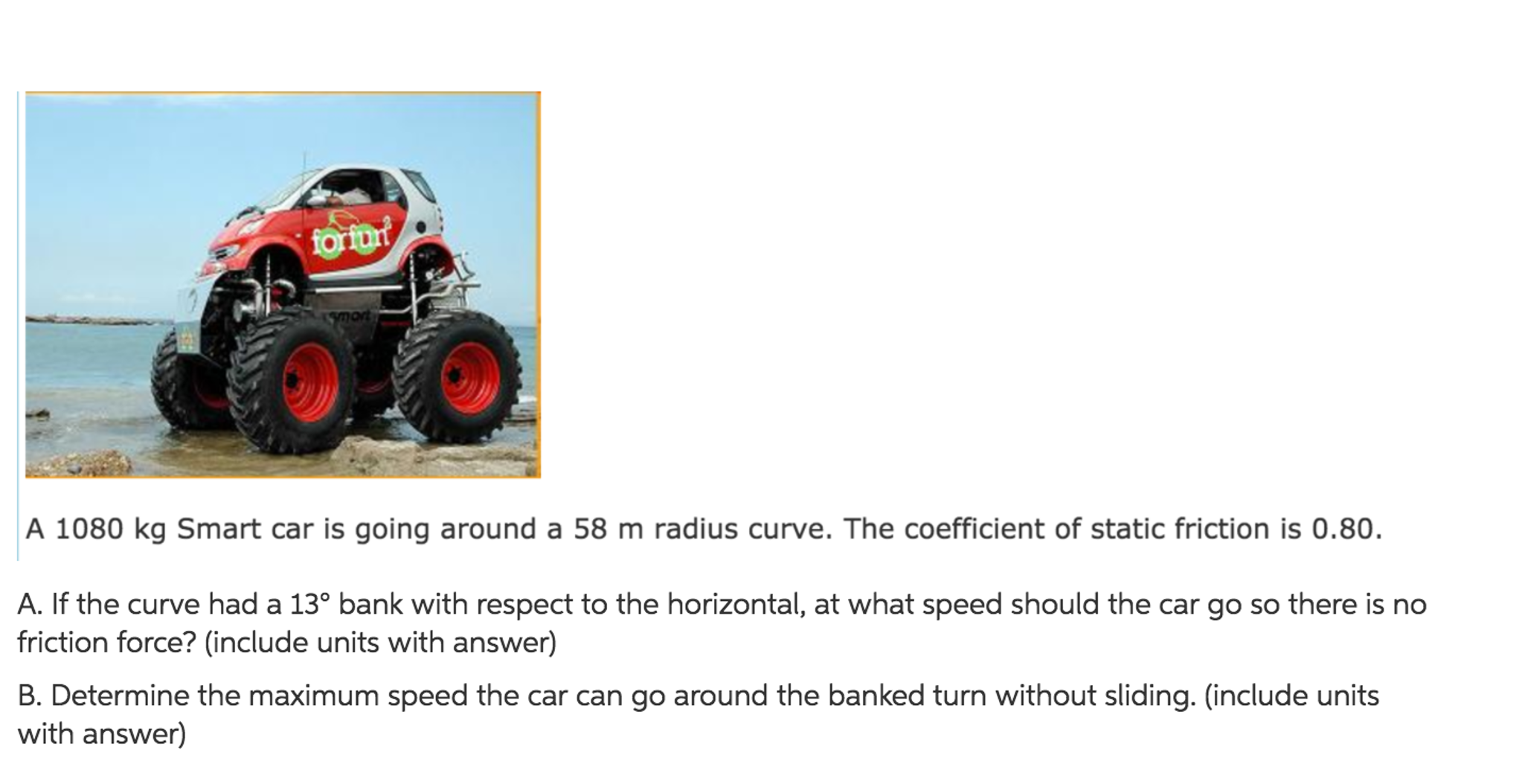A 1080 kg Smart car is going around a 58 m radius curve. The coefficient of static friction is 0.80. If the curve had a 13degree bank with respect to the horizontal, at what speed should the cargo so there is no friction force? (include units with answer) Determine the maximum speed the car can go around the banked turn without sliding, (include units with answer)

• ### A car of mass M = 1300 kg traveling at 65.0 km/hour enters a banked turn...

A car of mass M = 1300 kg traveling at 65.0 km/hour enters a banked turn covered with ice. The road is banked at an angle θ, and there is no friction between the road and the car's tires as shown in (Figure 1) . Use g = 9.80 m/s2 throughout this problem. r= 91.43 m. Now, suppose that the curve is level (θ=0) and that the ice has melted, so that there is a coefficient of static friction μ...

• ### please answer 2 questions pleaeeee #o: A 600-kg car traveling at 24.5 m/s is going around...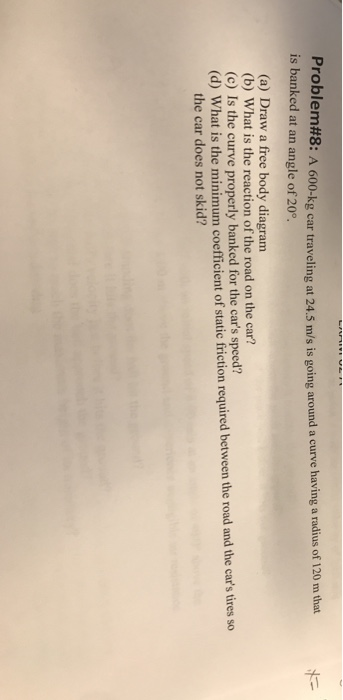please answer 2 questions pleaeeee #o: A 600-kg car traveling at 24.5 m/s is going around a curve having a radius of 120 m that is banked at an angle of 20°. (a) Draw a free body diagram (b) What is the reaction of the road on the car? (c) Is the curve properly banked for the car's speed? (d) What is the minimum coefficient of static friction required between the road and the car's tires so the car does...